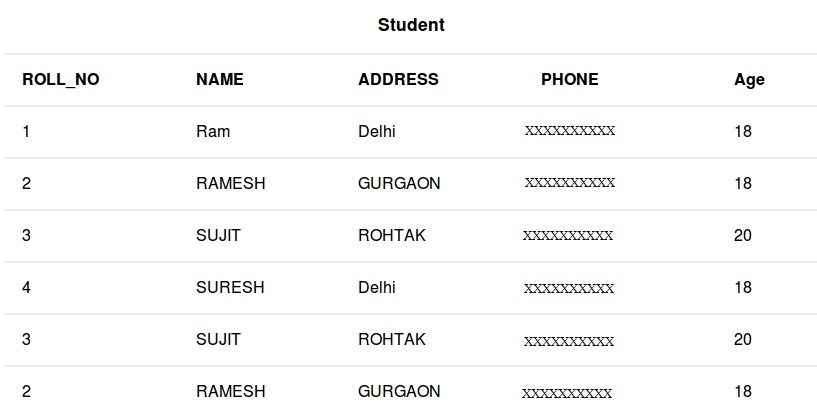Open in App
Not now

# SQL AND and OR operators

• Difficulty Level : Basic
• Last Updated : 04 Oct, 2021

In SQL, the AND & OR operators are used for filtering the data and getting precise results based on conditions. The SQL AND & OR operators are also used to combine multiple conditions. These two operators can be combined to test for multiple conditions in a SELECT, INSERT, UPDATE, or DELETE statement.

When combining these conditions, it is important to use parentheses so that the database knows what order to evaluate each condition.

• The AND and OR operators are used with the WHERE clause.
• These two operators are called conjunctive operators.

### AND Operator:

This operator displays only those records where both the conditions condition1 and condition2 evaluates to True.

Syntax:

```SELECT * FROM table_name WHERE condition1 AND condition2 and ...conditionN;

table_name: name of the table
condition1,2,..N : first condition, second condition and so on```

### OR Operator:

This operator displays the records where either one of the conditions condition1 and condition2 evaluates to True. That is, either condition1 is True or condition2 is True.
Syntax:

```SELECT * FROM table_name WHERE condition1 OR condition2 OR... conditionN;

table_name: name of the table
condition1,2,..N : first condition, second condition and so on
```

Now, we consider a table database to demonstrate AND & OR operators with multiple cases:If suppose we want to fetch all the records from the Student table where Age is 18 and ADDRESS is Delhi. then the query will be:

Query:

`SELECT * FROM Student WHERE Age = 18 AND ADDRESS = 'Delhi';`

Output:

Take another example, to fetch all the records from the Student table where NAME is Ram and Age is 18.

Query:

`SELECT * FROM Student WHERE Age = 18 AND NAME = 'Ram';`

Output:

To fetch all the records from the Student table where NAME is Ram or NAME is SUJIT.

Query:

`SELECT * FROM Student WHERE NAME = 'Ram' OR NAME = 'SUJIT';`

Output:

To fetch all the records from the Student table where NAME is Ram or Age is 20.

Query:

`SELECT * FROM Student WHERE NAME = 'Ram' OR Age = 20;`

Output:

### Combining AND and OR:

We can combine AND and OR operators in the below manner to write complex queries.

Syntax:

`SELECT * FROM table_name WHERE condition1 AND (condition2 OR condition3);`

Take an example to fetch all the records from the Student table where Age is 18 NAME is Ram or RAMESH.

Query:

`SELECT * FROM Student WHERE Age = 18 AND (NAME = 'Ram' OR NAME = 'RAMESH');`

Output:

My Personal Notes arrow_drop_up